Home

The square

TYPE A SQUARE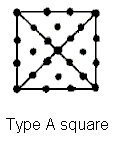(1) The square has 25 yods (five corners, 20 hexagonal yods). The 25th odd integer is 49, the Godname number of Yesod, which is thus encoded in the square. It also symbolizes the 25 spatial dimensions of bosonic strings, the central yod symbolizing their longitudinal dimension (direction along string) and the surrounding 24 yods symbolizing their 24 transverse dimensions (directions perpendicular to string). The latter divide into the eight yods (four corners & four centres of tetractyses) that symbolize the eight transverse dimensions predicted by superstring theory and the (8+8=16) hexagonal yods on sides of tetractyses that symbolize the extra 16 transverse dimensions predicted by string theory.

The sum of the first 12 integers that can be assigned to the yods on the boundary of a square:

 1 2 3 4 12 5 11 6 10 9 8 7

is 78. This is the dimension of E6, the rank-6 exceptional subgroup of E8. The sum of the squares of these integers is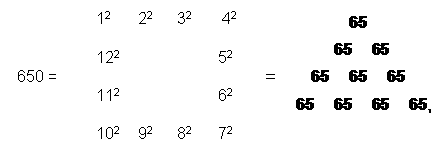where 65 is the number of ADONAI, the Godname of Malkuth. Since the latter prescribes the 10-tree representing the 10-dimensional space-time of superstrings, we see that the square encodes the 25 spatial dimensions of bosonic strings, the group E6 and the number of ADONAI prescribing the dimensionality of superstring space-time. According to #17 in Wonders of sacred geometry/Miscellaneous wonders, there are 650 yods other than their corners associated with each set of seven enfolded, Type B polygons making up the inner form of the Tree of Life. Therefore, this yod population of an example of sacred geometry is embodied arithmetically in the Type A square through the squares of the integers 1–12 that can be assigned to its sides.

(2) Sum of first 25 integers = 325 = ¼(15 + 25 + 35 + 45), i.e., 325 is the arithmetic mean of the fifth powers of 1, 2, 3 & 4 (notice that 25 is the mean of their third powers: 25 = ¼(13 + 23 + 33 + 43). The sum of the 50 integers assigned to the 50 yods in two separate squares is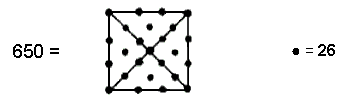The square therefore connects the number value 26 of YAHWEH and the number value 50 of ELOHIM, the Godnames of the second and third Sephiroth, to the yods in the seven enfolded polygons other than their corners. In fact, the sum of the numbers of the complete Godnames of the first four Sephiroth is 650: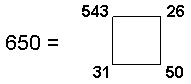Once again, the Tetrad reveals through the square — its geometrical symbol — its power to express parameters of sacred geometry.

(3) The first 25 integers consist of the first 13 odd integers and the first 12 even integers. Given that there are 13 yods inside the Type A square and 12 yods on its boundary, it is natural to assign the former integers to the 13 interior yods of the square (with the integer 1 at its centre) and the latter integers to the 12 yods on its boundary. The sum of the 12 odd integers surrounding its centre is 168. This is the number of Cholem Yesodoth, the Mundane Chakra of Malkuth, traditionally identified with the four elements of Earth, Water, Air and Fire. The interior of the Type A square embodies the structural parameter of superstrings. The sum of the even integers on the boundary is 156, which is the sum of the values of all possible combinations of the letters of YHVH, the Godname of Chokmah:

Y = 5, H = 5, V = 6.

Y + H + V =                   21
YH + YV + HH + HV =  52
YHH + YVH + HVH =    57
YHVH =                         26
Total = 156

156 is the 155th integer after 1, where 155 is the number value of ADONAI MELEKH, the complete Godname of Malkuth. It is also the number of SLs that emanate from the first Sephirah of Construction of the 26th Tree. The boundary of the square therefore arithmetically embodies a number that, in the context of CTOL, locates the counterpart in higher realms of consciousness of time, the 26th dimension, namely, the 26th Tree, whilst its interior embodies a structural parameter of 26-dimensional strings. This is a remarkable property of the tetractys-divided square.

(4) Sum of squares of the first 25 integers = 5525 = 85×65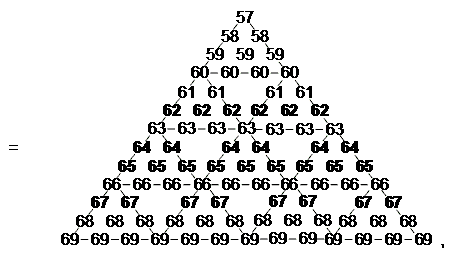where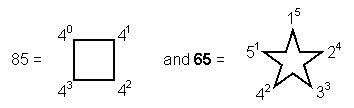.

The central yod of a tetractys symbolizes Malkuth, so it is remarkable that a 2nd-order tetractys array of consecutive integers with the Godname number 65 of Malkuth at its centre generates the number 5525. The sum of the squares of the internal integers assigned to the square is

12 + 32 + 52 +… + 252 = 2925 = 45×65.

This is the sum of the 45 integers on the boundary of the tetractys array shown below with 65 once again at its centre: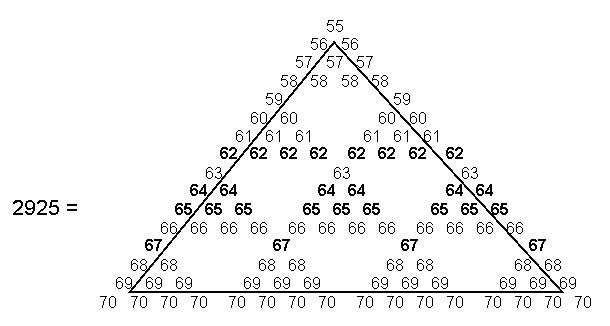Notice that the sum of the 100 (= 13 + 23 + 33 + 43) integers in this array is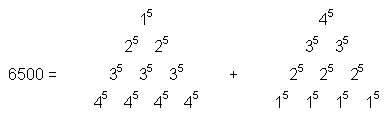.

The sum of the squares of the 12 boundary integers of the square is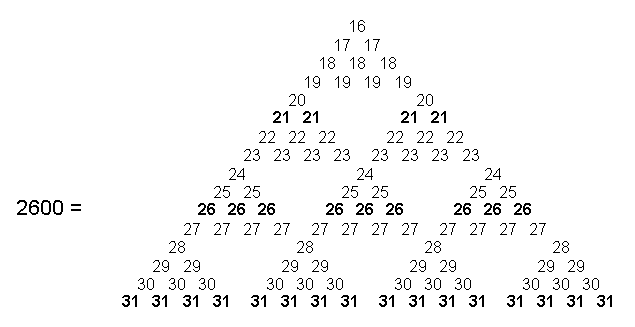Note that the sum of the 16 integers 31 at the base of this tetractys array with the number 26 of YAHWEH at its centre is 496, which is the number of Malkuth, the lowest Sephirah. 2600 is the sum of the first 50 odd integers after 1. It is, therefore, also prescribed by ELOHIM, the Godname of Binah with number value 50 — the Sephirah that heads the Pillar of Judgement opposite Chokmah at the top of the Pillar of Mercy.

(5) Assigning the cubes of 1, 2, 3 & 4 to the 25 yods in a Type A square generates the dimension 248 of the rank-8, exceptional Lie group E8 used in superstring theory: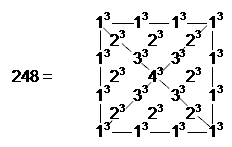This number has the remarkable property: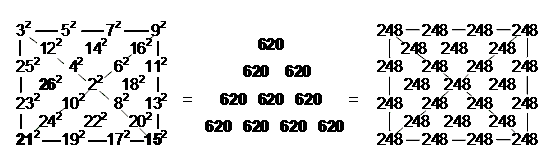Starting with the Pythagorean Tetrad 22 = 4 at the centre of the square, the sum of the 25 consecutive squares 22, 32262 generates the number 620 of Kether and is the same as the sum of the numbers 248 assigned to each yod of the four tetractyses composing the square. This illustrates how the square encodes not only the 25 spatial dimensions of bosonic strings and the dimension 248 of E8 but also the number of Kether.

(6) The Type A square displays another beautiful property of the Pythagorean Tetrad, Decad and integers 1, 2, 3 & 4: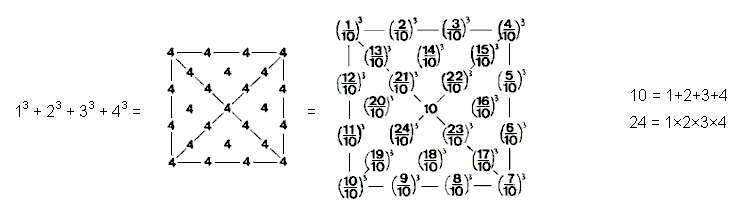(7) The Godname number of Binah has an analogous representation: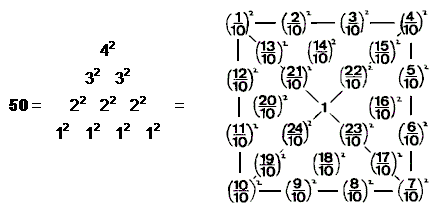(8) Two separate Type A squares have 50 yods, of which

 4 4 4 40 = 4 4 4 4 4 4 4

are hexagonal. Two enfolded Type A squares have 46 yods (the number of yods in a Type B triangle), of which 38 are hexagonal (the number of yods in two Type A triangles). 36 hexagonal yods are outside their shared edge.

TYPE B SQUARE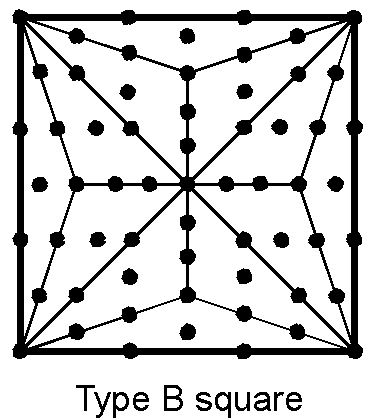(1) Number of yods = 61 = number of AIN, the ABSOLUTE. This number is the ratio: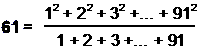where 91 is the number of Trees of Life in CTOL. 61 is the 31st odd integer. The Type B square has 49 internal yods and 52 hexagonal yods, where 52 is the 26th even integer.
(
2) Sum of first 61 integers = 1891 = 61×31, i.e., 31 is the arithmetic mean of the first 61 integers. Hence, the Type B square arithmetically encodes the Godname number of Chesed as well as that of Yesod.

(3) Assigning integers 1–61 to the yods of the square, with number 1 assigned to its centre, the sum of the 49 internal integers is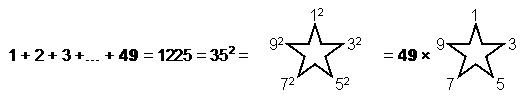.

Sum of 12 boundary integers = 50 + 51 + 52 +... + 61 = 666, which is the 36th triangular number.

(4) Sum of squares of first 61 integers = 12 + 22 +... + 612 = 77531 = 61×31×41, where 41 = 15 + 26.

Sum of squares of internal integers = 12 + 22 +... + 492 = 40425 = 49×15×55, where 55 is the tenth triangular number.

Sum of squares of boundary integers = 502 + 512 +... + 612 = 37106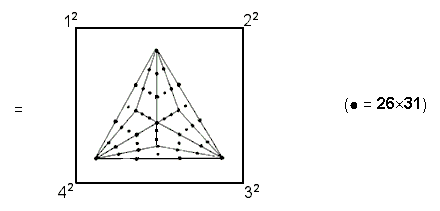This representation contains 50 integers, showing how YAHWEH, ELOHIM and EL prescribe this arithmetic property of the square.

(5) Number of hexagonal yods = 52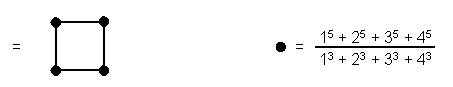The square has 50 hexagonal yods outside the root edge. Two squares have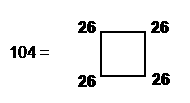hexagonal yods. Two enfolded squares have 100 (= 13 + 23 + 33 + 43) hexagonal yods outside the root edge. These properties show the defining role of the Tetrad.

TYPE A + TYPE B SQUARES
(1) Number of yods in two separate squares = 86 = 50 + 36. An enfolded pair of squares has 78 yods outside root edge. Hence, these two types of tetractys-divided square encode the superstring gauge group E6, whose dimension is 78.
(2) Number of hexagonal yods = 72 (separate), = 70 (enfolded). Two enfolded squares therefore encode the number 70 representing both the number of corners of the (7+7) enfolded polygons of the inner Tree of Life and the number of yods in its outer form when its 16 triangles are turned into tetractyses.

TYPE C SQUARE

A Type C square is one whose sectors are Type B triangles: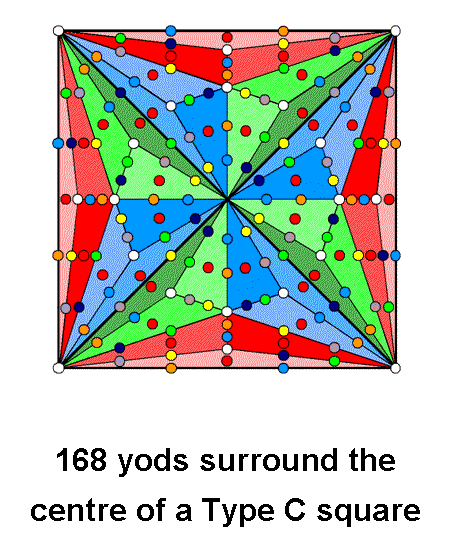Surrounding its centre are 168 yods. In accordance with the Tetrad Principle, because this square not only symbolizes the Tetrad but is the fourth in the sequence:

square→Type A square→Type B square→Type C square, etc,

the Tetrad determines the basic structural parameter 168 of the subquark state of the E8×E8 heterotic superstring.

The next example illustrates this principle as well because the square embodies one of the basic dynamical parameters of superstrings, namely, the dimension of E8.

SQUARE WITH 2ND-ORDER TETRACTYSES AS SECTORS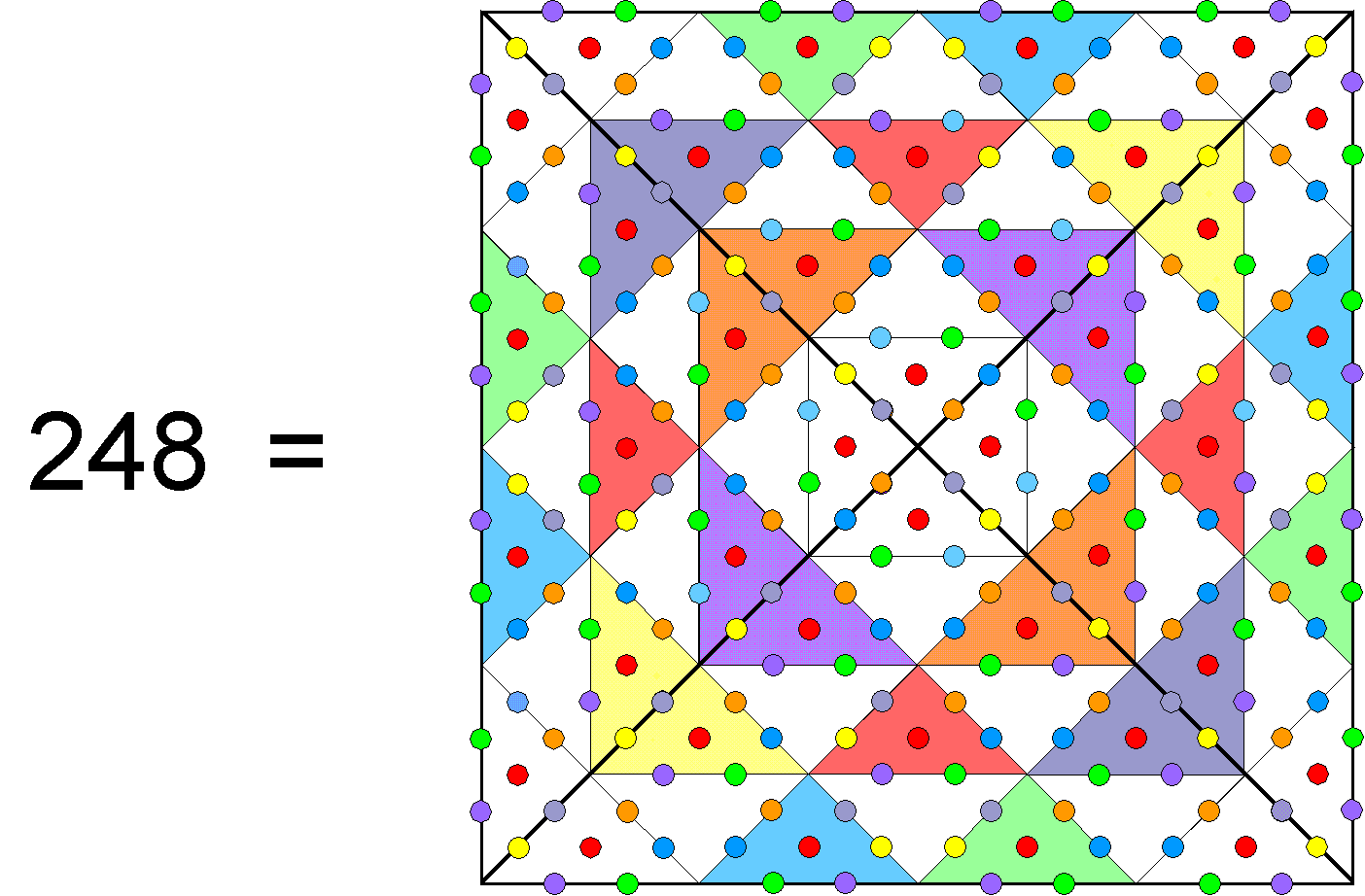A 2nd-order tetractys has 85 yods (see here). They comprise 15 corners of ten 1st-order tetractyses and 70 hexagonal yods. A square whose sectors are 2nd-order tetractyses has 288 yods surrounding its centre, where

 10 21 21 288 = 11 + 22 + 33 + 44 = 32 32 32 43 43 43 43 .

The 288 yods comprise 40 corners, where

 4 4 4 40 = 4×10 = 4(1+2+3=4) = 4 + 8 + 12 + 16 = 4 4 4 4 4 4 4 ,

and 248 hexagonal yods. Embodied in the square is not only the number 25 signifying the 25 spatial dimensions of spinless strings but also the dimension 248 of E8, the rank-8, exceptional Lie group at the heart of E8×E8 heterotic superstring theory. Each coloured hexagonal yod in the picture above symbolizes one of the 248 gauge bosons that transmit the unified force between superstrings of ordinary matter. EHYEH ("I am"), the Godname of Kether with number value 21, prescribes this geometrical representation of the archetypal number 248 because its 40 tetractyses have 41 corners and 41 is the 21st odd integer. It is discussed further in #9 in Superstrings as sacred geometry/Tree of Life.

Home